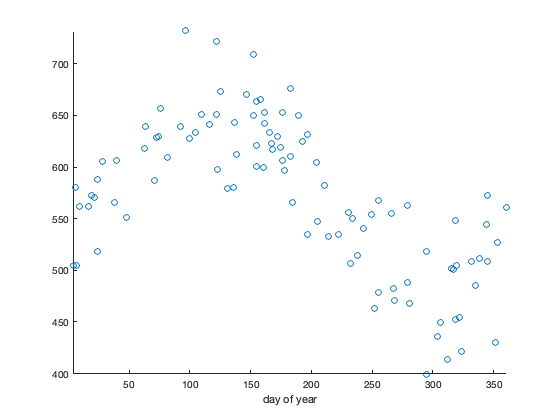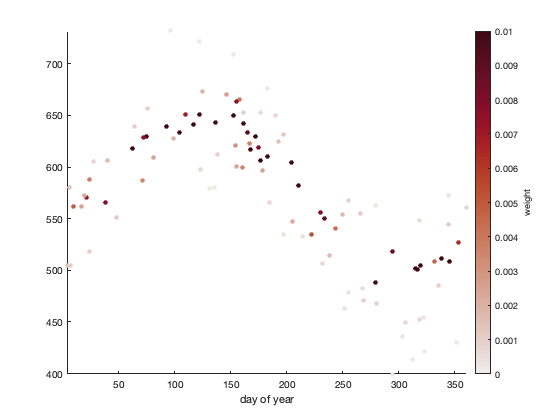# sinefit documentation

sinefit fits a least-squares estimate of a sinusoid to time series data that have a periodicity of 1 year.

For related functions, see sineval and sinefit_bootstrap documentation.

Back to Climate Data Tools Contents

## Syntax

```ft = sinefit(t,y)
ft = sinefit(...,'weight',weights)
ft = sinefit(...,'terms',TermOption)
[ft,rmse] = sinefit(...)```

## Description

ft = sinefit(t,y) fits a sinusoid with a periodicity of 1 year to input data y collected at times t. Input times can be in datenum, datetime, or datestr format, and do not need to be sampled at regular intervals. The output ft contains the coefficients of the terms in the calculation described below.

ft = sinefit(...,'weight',w) applies weighting to each of the observations y. For example, if formal errors err are associated with y, you might let w = 1./err.^2. By default, w = ones(size(y)).

ft = sinefit(...,'terms',TermOption) specifies which terms are calculated in the sinusoid fit. TermOption can be 2, 3, 4, or 5:

• 2: ft = [A doy_max] where A is the amplitude of the sinusoid, and doy_max is the day of year corresponding to the maximum value of the sinusoid. The default TermOption is 2.
• 3: ft = [A doy_max C] also estimates C, a constant offset. Solving for adds processing time, so you may prefer to estimate C on your own simply as the mean of the input y. However, if you can't assume C=mean(y), you may prefer this three-term solution.
• 4: ft = [A doy_max C trend] also estimates a linear trend over the entire time series in units of y per year. Again, simultaneously solving for four terms will be much more computationally expensive than solving for two yerms, so you may prefer to estimate the trend on your own with polyfit, then calculate the two-term sine fit on your detrended data.
• 5: ft = [A doy_max C trend quadratic_term] also includes a quadratic term in the solution, but this is experimental for now, because fitting a polynomial to dates referenced to year zero tends to be scaled poorly.

[ft,rmse] = sinefit(...) returns the root-mean-square error of the residuals of y after removing the sinusoidal fit. This is one measure of how well the sinusoid fits the data, but for a more in-depth understanding of the uncertainties, including uncertainties in the timing, see sinefit_bootstrap.

## Example 1a: Fit a sinusoid to toy data

In this example we'll make up some data with known parameters, then use sinefit to fit a sinusoid to the data. Let's assume you have 100 measurements taken between Jan 1, 2005 and today. Use sineval to generate a sinudoid of 75 unit amplitude, having a maximum value on day 123 of each year (July 5), and a long-term linear trend of -2.2 units per year. Note that in sineval we'll also have to enter a somewhat strange value of 5e3, which is just the y-intercept at year zero, but is not very meaningful to us in the 21st century.

To make it more of a challenge for sinefit, we'll also add gaussian noise that has a standard deviation of 36 units. (This is an appreciable amount of noise relative to the 75 unit amplitude of the sinusoid.)

```% 100 random dates between January 1, 2005 and today:
t = datenum('jan 1, 2005') + (now-datenum('jan 1, 2005'))*rand(100,1);

% Sinusoid of amplitude 75; max on day 123 (July 5); trend -2.2 units/year:
sine_parameters = [75 123 5e3 -2.2];
err = 36*randn(size(t)); % random error
y = sineval([75 123 5e3 -2.2],t) + err;  % sinusoid + noise

% Plot the 100 data points:
figure(1)
plot(t,y,'o')
axis tight % removes white space
box off    % removes frame
datetick('x','keeplimits') % formats x date labels
```Believe it or not, that dataset is sinusoidal. It's easier to see the sinusoidal nature if we use doy to plot all the data as a function of day of year:

```figure(2)
plot(doy(t),y,'o')

axis tight
box off
xlabel 'day of year'
```In this toy dataset, we know we should have a sinusoid with an amplitude of 75 units, a maximum value on day 123, and a long term trend of -2.2 units per year. And since we intentionally added 36 units of gaussian noise, we know the sinusoid should match the "measurements" to about an rms error of 36 units.

```[ft,ft_error] = sinefit(t,y,'terms',4)
```
```ft =
79.24        122.69       5867.81         -2.63
ft_error =
39.54
```

The numbers above tell us that sinefit has determined our 100 noisy datapoints are characterized by a sinusoid with an amplitude of ft(1) units, which we see is close to the prescribed value of 75, a maximum value near the 123rd day of the year, and a linear trend of about -2.2 units per year. The optional second output of the sinefit function tells us the sinusoid matches the "measurements" to a 1-sigma uncertainty given by ft_error, which is in line with the prescribed value of gaussian noise.

Here is the sinusoid fit to the data:

```% A daily array of times from the first datapoint to the last:
t_daily = min(t):max(t);

% The fit sinusoid at daily resolution:
y_daily = sineval(ft,t_daily);

figure(1) % goes back to the first figure
hold on
plot(t_daily,y_daily)
```## Example 1b: Specifying weights

Suppose you know the formal errors associated with each observation y. Some measurements have higher uncertainties associated with them than others. From the err vector we used above to prescribe noise, we'll weight each observation y like this:

```w = 1./err.^2;

figure
scatter(doy(t),y,25,w,'filled')
axis tight
xlabel 'day of year'
cb = colorbar;
ylabel(cb,'weight')
cmocean('amp')
caxis([0 0.01])
```The plot above shows that we give more weight to the values with lower errors associated with them. Now use sinefit with the specified weights:

```ft = sinefit(t,y,'weight',w,'terms',4)
```
```ft =
74.54        123.31       5627.04         -2.51
```

Comparing to the unweighted solution, you'll see that this version produces a slightly more accurate coefficients.

## Example 1c: More uncertainty quantification

For a more in-depth analysis of uncertainty in the sinusoid fit, check out sinefit_bootstrap, which can provide 1-sigma levels of uncertainty for each parameter like this (give it a few seconds):

```ftb = sinefit_bootstrap(t,y,'terms',4);

% 1 sigma uncertainty for each parameter:
std(ftb)
```
```ans =
6.09          3.84       2075.83          1.03
```

That tells us sinefit should be accurate to 1-sigma levels of about 5 for the amplitude (compare ft(1) to the prescribed amplitude to prove this to yourself); timing should be accurate within about 4 days; and the trend should be accurate within about 1 unit per year.

## Example 2: Fit a sinusoid to real sea ice data

Let's take a look at some real sea ice data from the NSIDC. I've packaged up a time series of sea ice extent in .mat format, and included in this File Exchange upload so you can follow along:

```% Load sample data:

% Plot raw data:
figure
plot(t,extent_N)
hold on
axis tight
box off
ylabel 'NH sea ice extent (10^6 km^2)'
```Quite clearly that data has some periodicity to it at the 1/yr frequency. How much does Northern Hemisphere sea ice extent vary at subannual timescales, when does it typically reach a maximum value, and how much is sea ice changing in terms of long-term trend?

```% Fit a sinusoid:
ft = sinefit(t,extent_N,'terms',4)
```
```ft =
4.41         66.84        125.30         -0.06
```

Similar to Example 1, the output of sinefit tells us Arctic sea ice tends to vary by about 4.41 million square kilometers each year, it reaches a maximum around day 66 (March 7) (see note below), and northern hemisphere sea ice has decreased by 60,000 square kilometers per year since 1978.

Here's the sinusoid fit plotted on top of the raw data:

```hold on
plot(t,sineval(ft,t))
legend('raw data','fit by sinefit')
```And zoom in a bit to see the structure:

```xlim([datetime(1995,1,1) datetime(2000,1,1)])
```## Note to users

One brief note related to the all the parameters estimated by sinefit: These parameters describe the best-fit sinusoid, but that does not necessarily mean they fully describe the behavior of the underlying data itself. For example, in terms of climatological average, Northern Hemisphere sea ice extent actually typically reaches a maximum around day 71 (March 12), whereas sinefit says the maximum value of the best-fit sinusoid occurs on day 66 (March 7). That's because the true behavior of sea ice extent is more complex than a simple sinusoid. In your work, be sure to consider the difference between true behavior and the 1/yr frequency component of the true behavior.

## Author Info

This function is part of the Climate Data Toolbox for Matlab. The sinefit, sineval, and sinefit_bootstrap functions as well as the supporting documentation were written by Chad A. Greene of the University of Texas Institute for Geophysics.